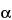Search USP29

INTERPRETATION OF CHROMATOGRAMS
Figure 1 represents a typical chromatographic separation of two substances, 1 and 2, where t1 and t2 are the respective retention times, h, h/2, and Wh/2 are the height, the half-height, and the width at half-height, respectively, for peak 1. W1 and W2 are the respective widths of peaks 1 and 2 at the baseline. Air peaks are a feature of gas chromatograms and correspond to the solvent front in liquid chromatography.
Figure 1.Chromatographic separation of two substances
Chromatographic retention times are characteristic of the compounds they represent but are not unique. Coincidence of retention times of a test and a reference substance can be used as a feature in construction of an identity profile but is insufficient on its own to establish identity. Absolute retention times of a given compound vary from one chromatogram to the next. Comparisons are normally made in terms of relative retention,, which is calculated by the equation:
in which t2 and t1 are the retention times, measured from the point of injection, of the test and reference substances, respectively, determined under identical experimental conditions on the same column, and ta is the retention time of a nonretained substance, such as methane in the case of gas chromatography.
In this and the following expressions, the corresponding retention volumes or linear separations on the chromatogram, both of which are directly proportional to retention time, may be substituted in the equations. Where the value of ta is small, RR may be estimated from the retention times measured from the point of injection (t2/t1).
The number of theoretical plates, N, is a measure of column efficiency. For Gaussian peaks, it is calculated by the equation:
in which t is the retention time of the substance and W is the width of the peak at its base, obtained by extrapolating the relatively straight sides of the peak to the baseline. Wh/2 is the peak width at half-height, obtained directly by electronic integrators. The value of N depends upon the substance being chromatographed as well as the operating conditions such as mobile phase or carrier gas flow rates and temperature, the quality of the packing, the uniformity of the packing within the column and, for capillary columns, the thickness of the stationary phase film, and the internal diameter and length of the column.
The separation of two components in a mixture, the resolution, R, is determined by the equation:
in which t2 and t1 are the retention times of the two components, and W2 and W1 are the corresponding widths at the bases of the peaks obtained by extrapolating the relatively straight sides of the peaks to the baseline.
Where electronic integrators are used, it may be convenient to determine the resolution, R, by the equation:
and to determine the number of theoretical plates, N, by the equation:
however, in the event of dispute, only equations based on peak width at baseline are to be used.
Peak areas and peak heights are usually proportional to the quantity of compound eluting. These are commonly measured by electronic integrators but may be determined by more classical approaches. Peak areas are generally used but may be less accurate if peak interference occurs. For manual measurements, the chart should be run faster than usual, or a comparator should be used to measure the width at half-height and the width at the base of the peak, to minimize error in these measurements. For accurate quantitative work, the components to be measured should be separated from any interfering components. Peak tailing and fronting and the measurement of peaks on solvent tails are to be avoided.
Chromatographic purity tests for drug raw materials are sometimes based on the determination of peaks due to impurities, expressed as a percentage of the area due to the drug peak. It is preferable, however, to compare impurity peaks to the chromatogram of a standard at a similar concentration. The standard may be the drug itself at a level corresponding to, for example, 0.5% impurity, or in the case of toxic or signal impurities, a standard of the impurity itself.